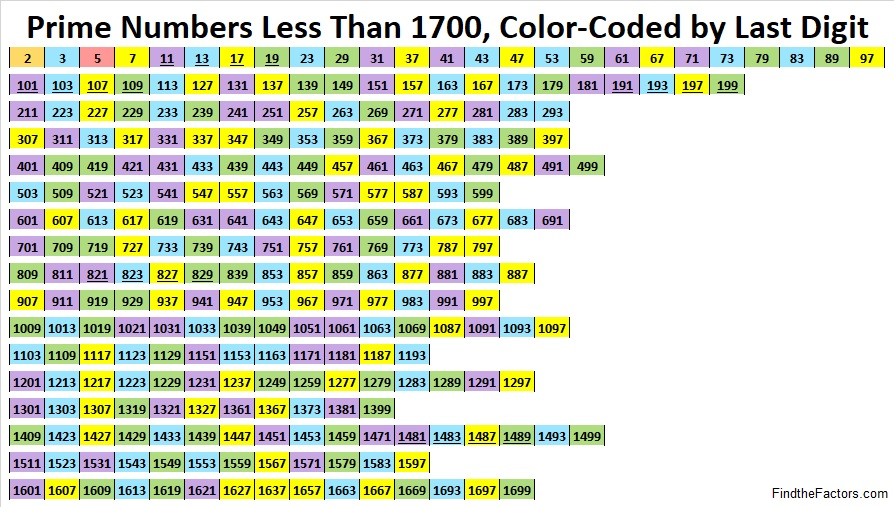# 1627 Color-Coded Prime Numbers

Contents

### Today’s Puzzle:

Study this color-coded chart of prime numbers. 1627 is the smallest prime number that begins something special. Can you figure out what that is?Also, why do you think I’ve underlined some of the other prime numbers on the list?

Memorizing which numbers are prime can be a big time-saver in mathematics. How many in a row can you recite without looking?

### Factors of 1627:

• 1627 is a prime number.
• Prime factorization: 1627 is prime.
• 1627 has no exponents greater than 1 in its prime factorization, so √1627 cannot be simplified.
• The exponent in the prime factorization is 1. Adding one to that exponent we get (1 + 1) = 2. Therefore 1627 has exactly 2 factors.
• The factors of 1627 are outlined with their factor pair partners in the graphic below.How do we know that 1627 is a prime number? If 1627 were not a prime number, then it would be divisible by at least one prime number less than or equal to √1627. Since 1627 cannot be divided evenly by 2, 3, 5, 7, 11, 13, 17, 19, 23, 29, 31, or 37, we know that 1627 is a prime number.

### More about the Number 1627:

1627 is the sum of two consecutive numbers:
813 + 814 = 1627.

1627 is also the difference of two consecutive squares:
814² – 813² = 1627.

What do you think of that?

This site uses Akismet to reduce spam. Learn how your comment data is processed.